Home > A2C > Chapter 9 > Lesson 9.2.3 > Problem9-105

9-105.
1.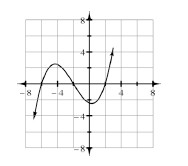In parts (a) through (d) below, for each polynomial function f(x), the graph of f(x) is shown. Based on this information, tell how many linear and quadratic factors the factored form of its equation should have and how many real and complex (non-real) solutions f(x) = 0 might have. (Assume a polynomial function of the lowest possible degree for each one.)

2. Example: f(x) at right will have three linear factors, therefore three real roots and no complex roots. Homework Help ✎

 a.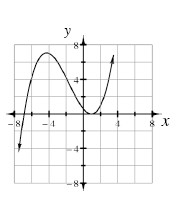b.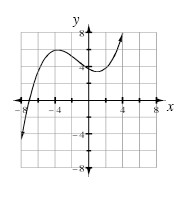c.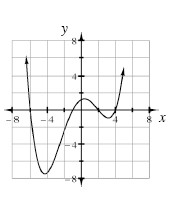d.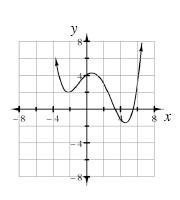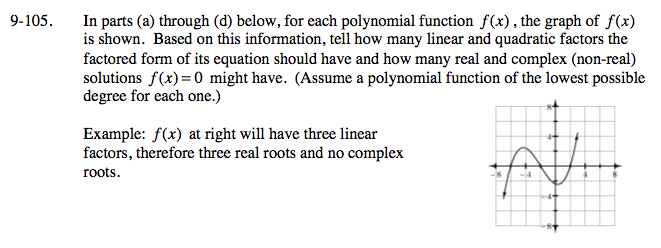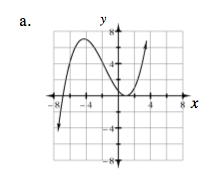There will be three linear factors (one is repeated), so the function will have two unique real roots and no complex roots.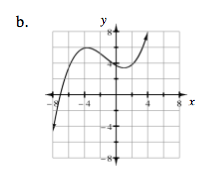There will be one linear factor and one quadratic factor, so the function will have one real root and two complex roots.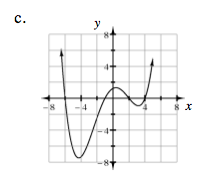There will be four linear factors, so the function will have four real roots.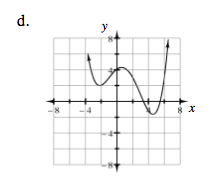There will be two linear factors and one quadratic factor, so the function will have two real roots and two complex roots.